Search
• Marek Vavrovic

# Linear regression analysis in Excel

Updated: Jul 12, 2020

In statistical modeling, regression analysis is used to estimate the relationships between two or more variables:

Dependent variable (aka criterion variable) is the main factor you are trying to understand and predict.

Independent variables (aka explanatory variables, or predictors) are the factors that might influence the dependent variable.

Regression analysis helps you understand how the dependent variable changes when one of the independent variables varies and allows to mathematically determine which of those variables really has an impact.

Technically, a regression analysis model is based on the sum of squares, which is a mathematical way to find the dispersion of data points. The goal of a model is to get the smallest possible sum of squares and draw a line that comes closest to the data.

In statistics, they differentiate between a simple and multiple linear regression. Simple linear regression models the relationship between a dependent variable and one independent variable using a linear function. If you use two or more explanatory variables to predict the dependent variable, you deal with multiple linear regression. If the dependent variable is modeled as a non-linear function because the data relationships do not follow a straight line, use nonlinear regression instead.

Linear regression equation

Mathematically, a linear regression is defined by this equation:

y = bx + a + ε

Where:

• x is an independent variable.

• y is a dependent variable.

• a is the Y-intercept, which is the expected mean value of y when all x variables are equal to 0. On a regression graph, it is the point where the line crosses the Y axis.

• b is the slope of a regression line, which is the rate of change for y as x changes.

• ε is the random error term, which is the difference between the actual value of a dependent variable and its predicted value.

The linear regression equation always has an error term because, in real life, predictors are never perfectly precise. However, some programs, including Excel, do the error term calculation behind the scenes. So, in Excel, you do linear regression using the least squaresmethod and seek coefficientsaandbsuch that:

y = bx + a

Example 1: Tips for serving

Let us assume that you are a small restaurant owner. Tips are a particularly important part of a waiter’s pay. Most of the time the pound amount of the tip is related to the amount of the total bill. As the restaurant owner you would like to develop a model that will allow you to make a prediction about what amount of tip to expect for any given bill amount. Therefore, one evening, you collect data for 6 meals. Unfortunately, when you begin to look at your data, you realize you only collected data for the tip amount and not the meal amount also. This is the best data you have. How might you predict the tip amount for future meals using only this data?With only one variable, and no other information, the best prediction for the measurement is the mean of the sample itself. The variability in the tip amounts can only be explained by the tips themselves.

The goal of simple linear regression it to create a linear model that minimizes the sum of squares of the residuals / errors (SSE).

Simple linear regression is a comparison of two models. One is where the independent variable does not even exist [use mean]. The other uses best fit regression line. If there is only one variable, the best prediction of other values is the mean of the dependent variable. The difference between the best fit line and the observed value is called the residual (or error). The residuals are squared and then added together to generate sum of squares residuals / errors, SSE. Simple linear regression is designed to find the best fitting line through the data that minimizes the SSE.

Example 2: Tips for serving

In the previous example we only had the tip data. In this one we also have the total bill. Now we can work with two variables. You want to know to what degree the tip amount can be predicted by the bill. The tip is the dependent variable; bill is the independent variable.

· Least squares criterion

min Σ(yi - ŷi)^2

yi = observed value of dependent variable (tip amount)

ŷi = estimated (predicted) value of the dependent variable (predicted tip amount)

The goal is to minimize the sum of the squared differences between the observed value for the dependent variable (yi) and the estimated / predicted value of the dependent variable (ŷi) that is provided by the regression line. Sum of the squared residuals.

Not only that, but the sum of the squared residuals should be much smaller than when we just used the dependent alone.

Prepare a chart to see if the data follow a linear pattern. Does the data seem to fall along a line? What is the correlation coefficient, r? In this case r=0.866. Is the relationship strong? In this case yes. The best-fit regression line will / must pass through the centroid.

Correlation =CORREL(array1,array2)

Slope =SPLOPE(known_ys,known_xs)

Y-intercept =INTERCEPT(known_ys,known_xs)ŷi = 0.1462x-0.8188

· Quick interpretation

For every £1 the bill amount (x) increases, we would expect the tip amount to increase by £0.1462 or about 15 pence. If the bill amount (x) is zero, then the expected / predicted tip amount is £ -0.8188 or negative 82 pence. Does this make sense? No. Intercept may or may not make sense in the real world.

In example1 with the dependent variable, the only sum of squares is due to error. Therefore, it is also the total and maximum sum of squares for the data under analysis.

SSE=120; SSE=SST; SST=120

With both the independent variable and dependent variable, the total sum of squares remains the same. But the error sum of squares will be reduced significantly. The difference between SST and SSE is due to regression, SSR.

SST=120; SSE=?; SST-SSE=SSRDependent variable and independent variable, sum of squared errors comparison: when we conducted the regression, the SSE decreased from 120 to 30.075. That is 30.075 of the sum of squares was explained or allocated to ERROR. Where did the other 89.925 go? The 89.925 is the sum of squares due to REGRESSION. SST = SSR + SSE; 120 = 89.925 + 30.075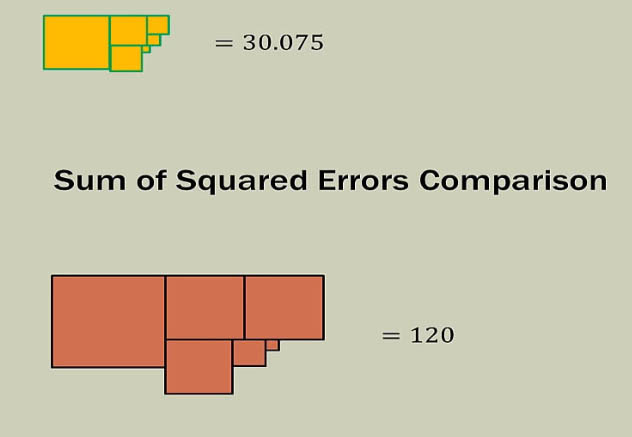Coefficient of determination

How well does the estimated regression equation fit our data? This is where regression begins to look a lot like ANOVA; the total sum of squares is partitioned or allocated to SSE and SSR. If SSR is large, it uses up more of SST and therefore SSE is smaller relative to SST. The coefficient of determination quantifies this ratio as a percentage.Coefficient of determination = r^2 = SSR/SST = 89.925/120=0.7493 or 74.93%

We can conclude that 74.93% of that sum of squares can be explained by using the estimated regression equation to predict the tip amount. The remainder is error.

black dashed line = mean

orange dots = observed values

purple dots = predicted valuesblue dots = observed values

orange dots = predicted values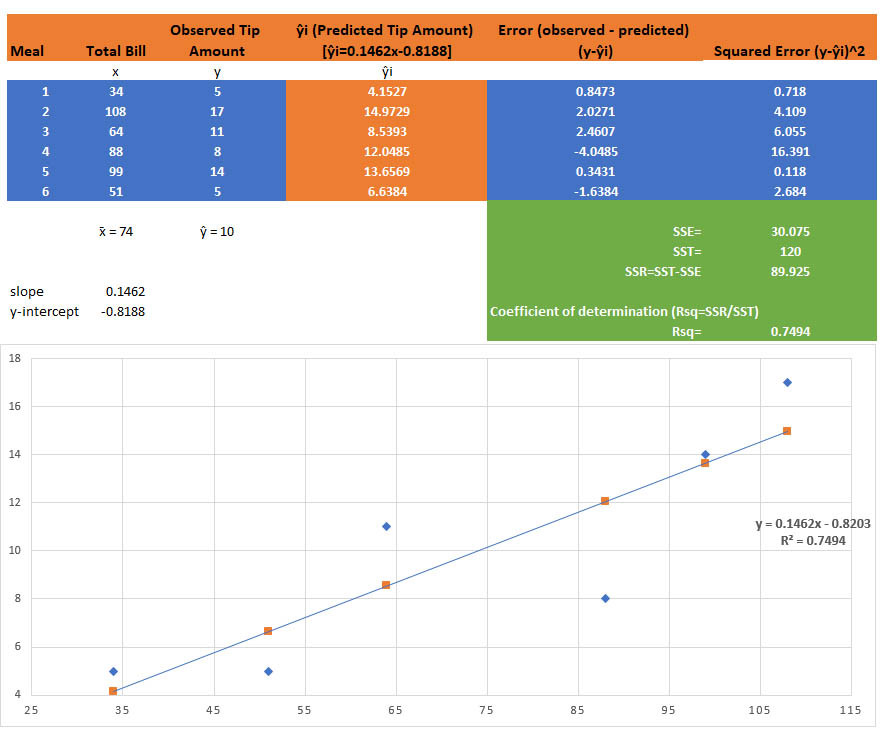Regression output, Multiple R in Excel: =CORREL(array1,array2)Regression equation: ŷi = 0.1462x-0.8203Mean Square Error / MS Residual

MSE is an estimate of the variance of the error. In other words, how spread out the data points are from the regression line. MSE is SSE divided by its degrees of freedom which is 2 because we are estimating the slope and intercept.

MSE [MS Residual] = SSE / n-2 = 30.075 / 6-2 = 7.5187

[n-2] we are using sample data. If we were using population data, we would just divide by N and it would simply be the average of the residuals.

Standard error of the estimate

The standard error of the estimate σ [Standard Error] is the standard deviation of the error term [unsquared]. It is the average distance an observation falls from the regression line in units of the dependent variable. Since the MSE [MS Residual] is s^2, the standard error is just the square root of MSE.So, the average distance of the data points from the fitted line is about £2.74

We calculate the errors, then we square them.Interval for the Slope

How much variance in the dependent variable is explained by the model / independent variable? For this we look at the value of Adjusted R Square. Does a statistically significant linear relationship exist between the independent and dependent variable?

Is the overall F-test or t-test (in simple regression these are the same thing) significant? Can we reject the null hypothesis that the slope b1 of the regression line is ZERO? Does the confidence interval for the slope b1 contain zero?

Linear regression contains many estimators:

· b1: the slope of the regression line

· bo: the intercept of the regression line on the y-axis

· centroid: the point that is the intersection of the mean of each variable (x̅,y̅), etc.Calculating the standard deviation of the slope.Confidence interval for slope.b1: point estimator for the slope

tα/2Sb1 : Margin of error

t: t value (df is n-2=4)

Sb1: standard deviation of the slope

t distribution with 4 degrees of freedom is 2.776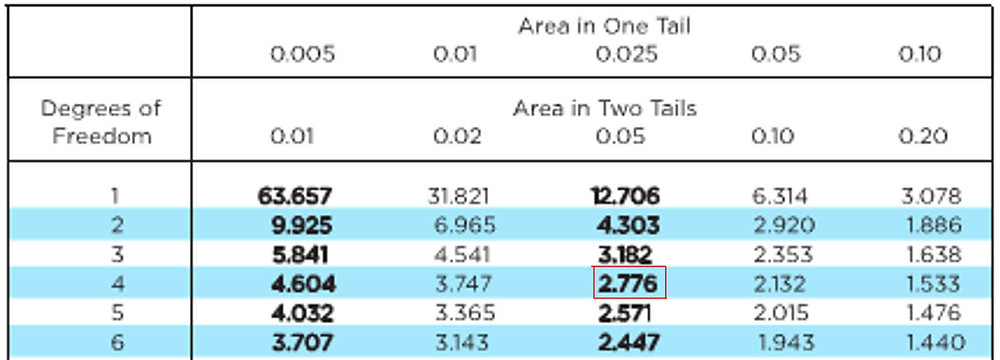We have equation like this and now we have to do some math.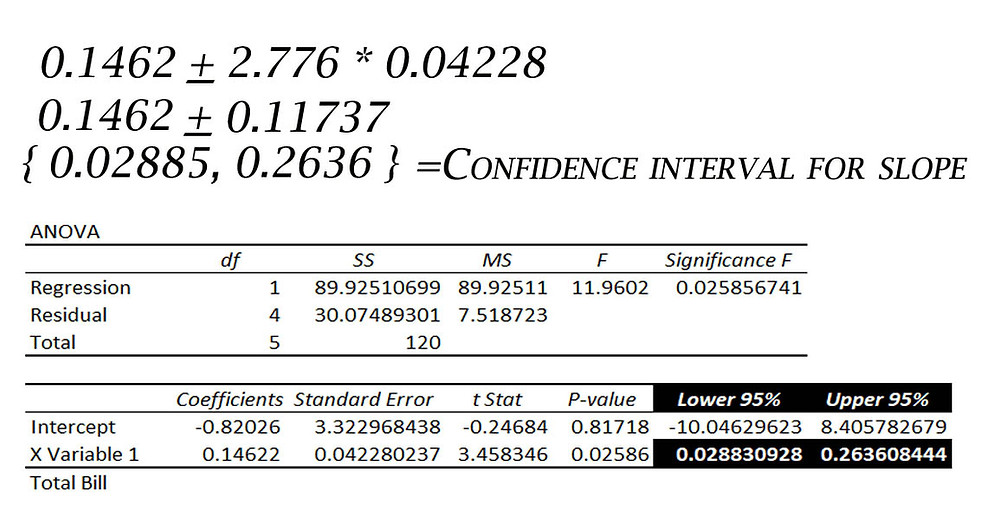We are 95% confident that the interval (0.02885, 0.2636) contains the true slope of the regression line. We can reject the null hypothesis that the slope is zero based on this 95% confidence interval.

Test statistic

t=b1/Sb1 [the slope of the regression line divided by standard deviation of the regression line]

t = 0.146219 / 0.04228 = 3.4584

we compare t Stat vs. t critical

3.4584 > 2.776 [3.4584 is outside the rejection region ]

p value is 0.0258 < 0.05

P value is low we can reject the null hypothesis

Confidence bands

Let’ s say that you are the server who has been keeping data about meals and tips amounts. A table then has a meal that cost £64. Based on the model we have been using, what pound amount might the server expect for a tip?

y̅ = -0.82025 + 0.14622 * TotalBill

y̅ = -0.82025 + 0.14622 * 64

y̅ = 8.5378 = £8.54

But remember that the regression model is based off a sample and therefore the statistics generated are estimates. Another server would almost certainly have different data and therefore a different model would be generated. Regression is not deterministic. For a meal that cost £64, a tip of £8.54 is just one of many possible tips. We can ask ourselves, what is the average tip for a £64 meal? To answer this question, we will go back to sampling distributions. We can not know an exact average mean tip, so we will generate a confidence interval for the mean tip amount when bill is £64.At a meal amount of £64, there is a distribution of mean tip amounts. We are going to calculate the 95% confidence interval. That is the dark line that spans the shaded band.Confidence interval for y̅ͯWhen xͯ = 64

y̅ͯ = -0.820257 + 0.1462197 * TotalBill

y̅ͯ = -0.820257 + 0.1462197 * 64

y̅ͯ = 8.537803

8.537803 ± 2.776445 * S y̅ͯ

Confidence interval for y̅ͯCalculating standard deviation of y̅ͯ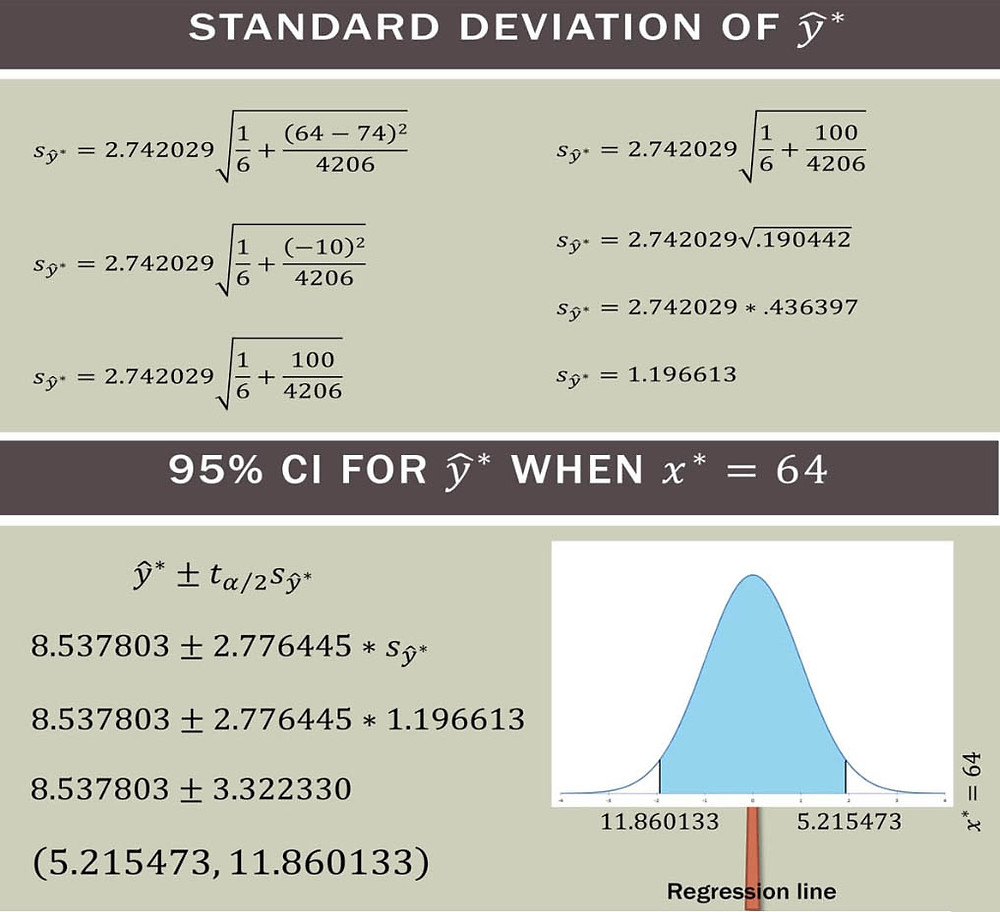The average tip for a £64 meal is between £5.22 and £ 11.86. This was calculated for 95% confidence interval.Example 3

A manager wants to find the relationship between the number of hours that a plant is operational in a week and weekly production. Here the independent variable x is hours of operation, and the dependent variable y is production volume. The production volume is dependent on how many hours of operation occurred at that plant during that week.

• Plot the data to see if there is a linear pattern.• Calculate means for production hours and volumne• Take the individual data points and subtract the mean from it• Multiply them together• · Square (x - x̅) . This is the denominator for the equation.• Calculate the slope for the regression equation• Calculate intercept• We got back the regression equation• · The manager wants to produce 125 units per week. How many hours they should run per week?• Output from excel## Recent Posts

See All

#### Index

Information functions Filter functions Table manipulation functions Grouping and summarizing Time intelligence functions OPENINGBALANCEYEAR OPENINGBALANCEYEAR(<Expression>,<Dates> [,<Filter>][,<YearEn